# Force facts for kids

Kids Encyclopedia Facts

In physics, a force is an interaction between objects. It is called an interaction because if one object acts on another, its action is matched by a reaction from the other object. This idea is known as Newton's third law, where action and reaction are "equal and opposite" (matched). The objects are just the things the force acts between. Different forces act between different sorts of object. For example, gravity acts between objects with mass, like the sun and the earth. Another example is electromagnetic force, which acts between objects with charge, like an electron and the nucleus of an atom. Gravity and electromagnetic force are two examples of forces.

A force changes the state of an object (some physical quantity changes) or, strictly, the states of two objects, since the force is an interaction. For example, a force causes an affected object to be pushed or pulled in a certain direction. This changes the object's momentum. Forces cause objects to accelerate, add to the object's overall pressure, change direction, or change shape. The strength of a force is measured in Newtons (N). There are four fundamental forces in physics.

A force is always a push, pull, or a twist, and it affects objects by pushing them up, pulling them down, pushing them to a side, or by changing their motion or shape in some other way.

## Newton's Second Law

According to Newton's Second Law of Motion, the formula for finding force is: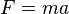$F = ma$

where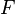$F$ is the force,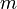$m$ is the mass of an object,
and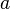$a$ is the acceleration of the object.

This formula says that when there is a force on an object then it will move faster and faster. If the force is weak and the object is heavy, then it will take a long time to increase the speed very much, but if the force is strong and the object is light, then it will move a lot faster very quickly.

### Weight

Gravity is an acceleration. Everything that has a mass is being pulled toward the Earth because of that acceleration. This pull is a force called weight.

One can take the equation above and change$a$ to the standard gravity g, then a formula about the gravity on earth can be found: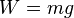$W = mg$

where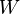$W$ is the weight of an object,$m$ is the mass of an object,
and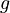$g$ is the acceleration due to gravity at sea level. It is about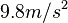$9.8m/s^2$.

This formula says that when you know the mass of an object, then you can calculate how much force there is on the object because of gravity. You must be on earth to use this formula. If you are on the moon or another planet, then you can use the formula but g will be different.

Force is a vector, so it can be stronger or weaker and it can also point in different directions. Gravity always points down into the ground (if you are not in space).

## Gravitational force

Another equation that says something about gravity is: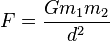${F}=\frac{Gm_{1}m_{2}}{d^2}$$F$ is force;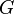$G$ is the gravitational constant, which is used to show how gravity accelerates an object;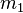$m_{1}$ is the mass of one object;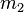$m_{2}$ is the mass of the second object; and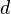$d$ is the distance between the objects.

This equation is used to calculate how the earth moves around the sun and how the moon moves around the earth. It is also used to calculate how other planets, stars and objects in space move around.

The equation says that if two objects are very heavy then there is a strong force between them because of gravity. If they are very far apart then the force is weaker.

## Images for kids

gd:NeartForce Facts for Kids. Kiddle Encyclopedia.